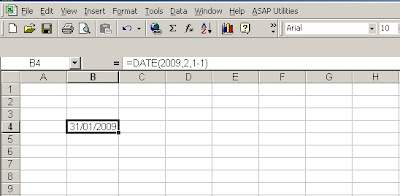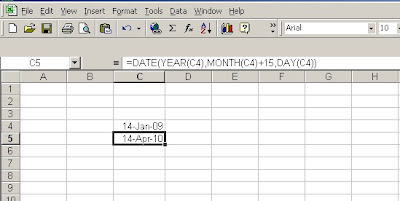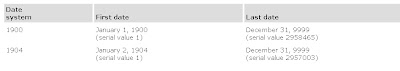## Friday, January 30, 2009

### Excel Vlookup function - Entering column number

Many users find it difficult to count and enter column number in Excel vlookup formula. This looks more difficult when the number of columns in the data range are large and gets complecated in case some of columns are hidden. However Microsoft Excel has inbuilt feature by which it helps you to know the column number. At the time of selecting table array it shows you the number of columns selected. If you notice this at the time of selection, you actualy do not need to count the number of columns. This works even if you have hidden columns in your data. Pls see video for further explanation.

## Saturday, January 24, 2009

### Entering last day of the month in Excel

Entering a specific date in excel is easy as you know what to enter, however if you are asked to enter last date of the month it becomes little tricky as last date of the month varies between 28 to 31 depending on what year and month it is.

However Excel date mathematics makes it easy. What you need to do is enter the first day of next month and then reduce it by 1, you will get last day of previous month.

Look at following picture to understand it better.I have used date function to enter Feb 1, 2009 and then reduced the day by 1, other wise you can enter =Date(2009,2,0) also to get same result. after you have added above formula in Cell B4 you can use this formula to enter last day of next months in cell B5 =DATE(YEAR(B4),MONTH(B4)+2,0). You can further copy this down to get next month.## Tuesday, January 20, 2009

### Formating cells in Excel

Data entered in excel shows differently on your worksheet depending upon the cell format used by you.

January 19, 2009 which is stored by system as 39832 can shown as 19-01-2009 or 19-Jan-2009 or
19-Jan-09. There are many combination that can be achieved by changing the cell format for the cell containing that value.

Similarly you can add currency sign such as Rs / \$ / £ / ¥ , it can be any sign of your choice.

Not only this you can actually add color codes the numbers depending upon the value.

You can choose all these options from Format Cell Menu. You need to choose Number tab than category as per requirement of the format.Most tricky / dynamic category is Custom format at the end of the list. How to use this in more productive way will be covered in later posts on the specific subjects like custom formating dates, custom formating numbers etc in excel.

## Sunday, January 4, 2009

### Excel date mathematics

Microsoft Excel stores dates as sequential numbers known as serial values. Excel stores times as decimal fractions because time is considered a portion of a day.

Dates and times are values and, therefore, can be added, subtracted, and included in other calculations. Will tell you date mathematics formula.

How to Add / Subtract month from a date in Excel.
Adding / Subtracting a month from a particular date in excel looks tough as each month has different number of days. However it is very simple if you use following formula.
=DATE(YEAR(C4),MONTH(C4)+1,DAY(C4))

In this base date is available at cell C4 and we have added 1 to month value to increase it by one month. Similarly you can subtract required number of months from base date. See picture below for further understanding of it.Addition of more than 12 months will result into addition of year. See picture below:-Addition of 15 months have resulted into increase of one year and 3 months.

This will work for Year addition / subtraction also.

## Date Add , Date Function , excel date mathematics , Excel Date math , using date , excel date calculate , excel date calculation , excel date function , excel date functions

### How Microsoft Excel stores dates and times

Microsoft Excel stores dates as sequential numbers known as serial values. Excel stores times as decimal fractions because time is considered a portion of a day.

Dates and times are values and, therefore, can be added, subtracted, and included in other calculations. For example, to determine the difference between two dates, you can subtract one date from the other. You can view a date as a serial value and a time as a decimal fraction by changing the format of the cell that contains the date or time to General format.

A day equals 1, half a day is .5 and an hour time interval is therefore 1/24=0.041666667

Dates entered without a specified year are assumed to be in the current year

Dates entered with a 2 digit year are stored as pre-2000 dates if the year is between 30 and 99 or as post-2000 dates if the year is between 0 and 29.

Excel supports two date systems: the 1900 and 1904 date systems. The default date system for Microsoft Excel for Windows is 1900 and Microsoft Excel for the Macintosh uses 1904 date system.

Two different date systems got created as 1900 is not a leap year and design of early Macintosh computers did not support dates before Jan 1, 1904. 1900, although divisible by 4, wasn’t a leap year. By using 1904 as a base, they saved a few bytes code, and every byte counted when they tried to squeeze the entire MacOS into 64KiB of ROM…

The following table shows the first date and the last date for each date system and the serial value associated with each date.To change to the 1904 date system, click Options on the Tools menu, click the Calculation tab, and then select the 1904 date system check box. This setting is workbook specific, you can have two workbooks on the same system with 1900 and 1904 date system.

Be careful while changing the date system. The workbooks with actual dates recorded will also shift by 1462 days.

Similarly you may get into trouble when linking two workbooks set with two different date systems. in case you get struck with such situation you can correct this by adding or subtracting 1462 from one of the workbook as the case may be.

Suppose Book1 works on 1900 date system and book2 works on 1904 date system. If you are linking dates from 1904 to 1900 date system workbook, you will add 1462 to all the dates linked from 1904 date system. In a reverse scenario you will subtract 1462.